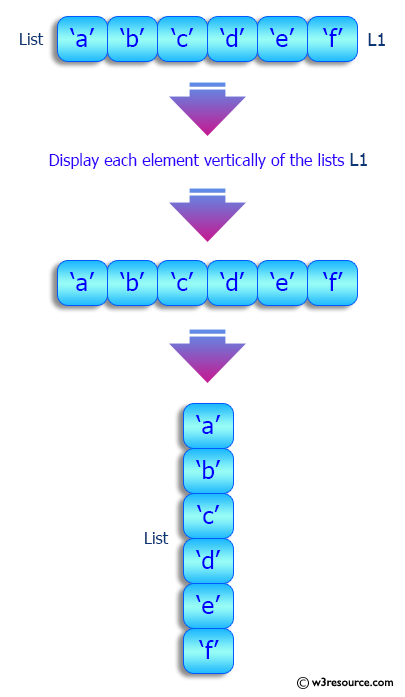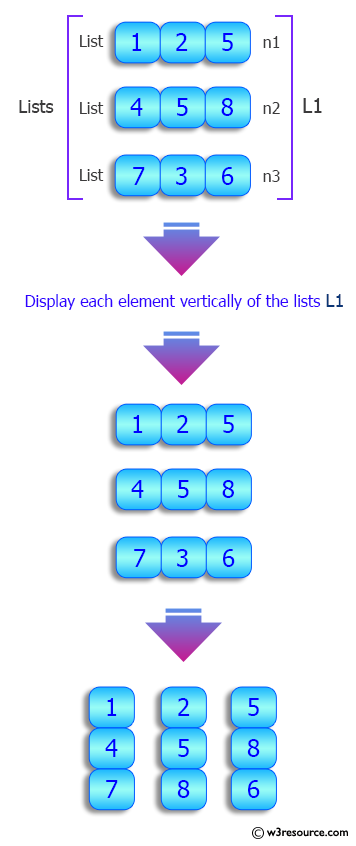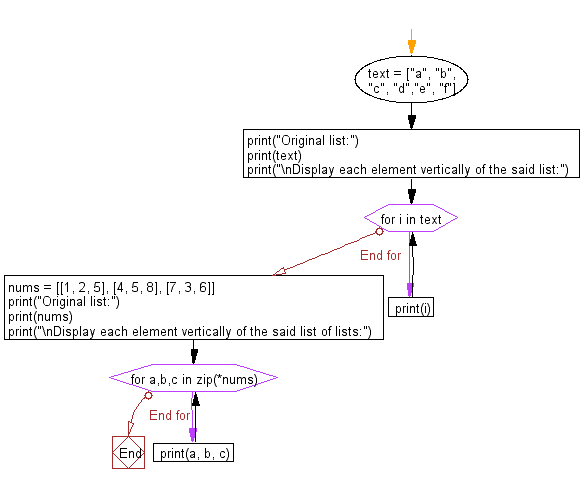﻿ Python: Display each element vertically of a given list, list of lists - w3resource# Python: Display each element vertically of a given list, list of lists

## Python List: Exercise - 168 with Solution

Write a Python program to display vertically each element of a given list, list of lists.

Sample Solution:

Python Code:

``````text = ["a", "b", "c", "d","e", "f"]
print("Original list:")
print(text)
print("\nDisplay each element vertically of the said list:")
for i in text:
print(i)
nums = [[1, 2, 5], [4, 5, 8], [7, 3, 6]]
print("Original list:")
print(nums)
print("\nDisplay each element vertically of the said list of lists:")
for a,b,c in zip(*nums):
print(a, b, c)
```
```

Sample Output:

```Original list:
['a', 'b', 'c', 'd', 'e', 'f']

Display each element vertically of the said list:
a
b
c
d
e
f
Original list:
[[1, 2, 5], [4, 5, 8], [7, 3, 6]]

Display each element vertically of the said list of lists:
1 4 7
2 5 3
5 8 6
```

Pictorial Presentation:Flowchart:## Visualize Python code execution:

The following tool visualize what the computer is doing step-by-step as it executes the said program:

Python Code Editor:

Have another way to solve this solution? Contribute your code (and comments) through Disqus.

What is the difficulty level of this exercise?

Test your Python skills with w3resource's quiz

﻿

## Python: Tips of the Day

Floor Division:

When we speak of division we normally mean (/) float division operator, this will give a precise result in float format with decimals.

For a rounded integer result there is (//) floor division operator in Python. Floor division will only give integer results that are round numbers.

```print(1000 // 300)
print(1000 / 300)```

Output:

```3
3.3333333333333335```